Ex 3.5

Chapter 3 Class 10 Pair of Linear Equations in Two Variables
Serial order wise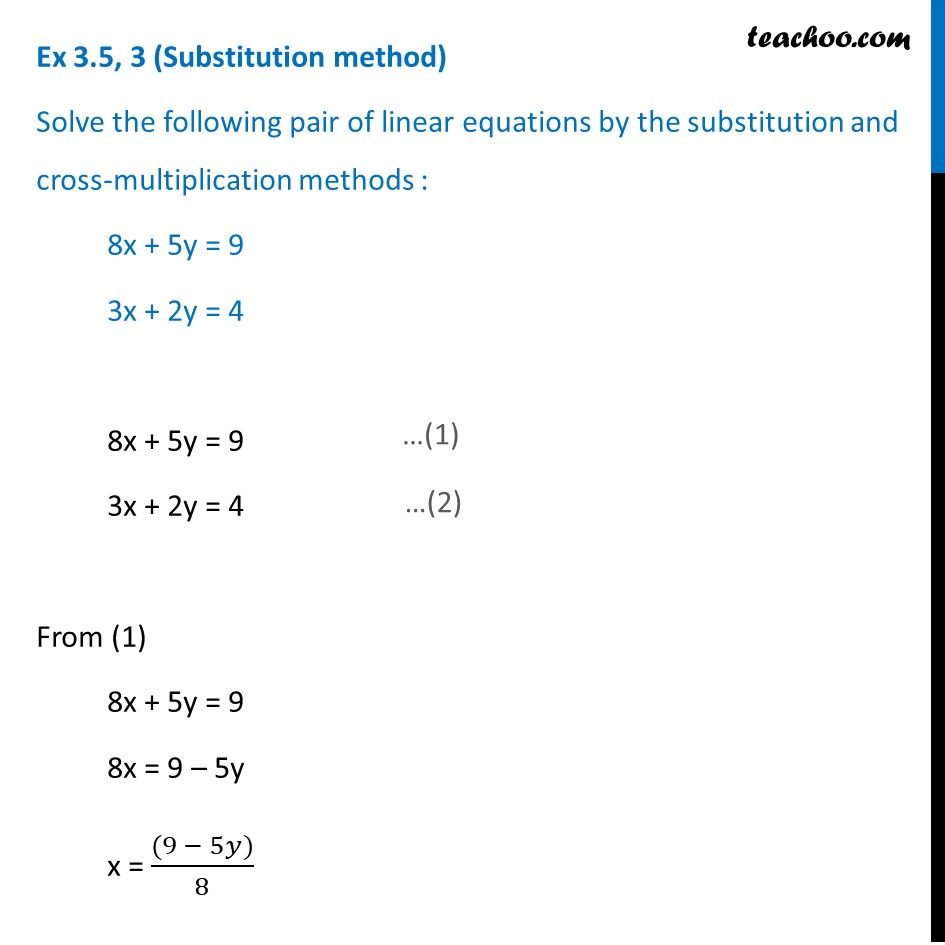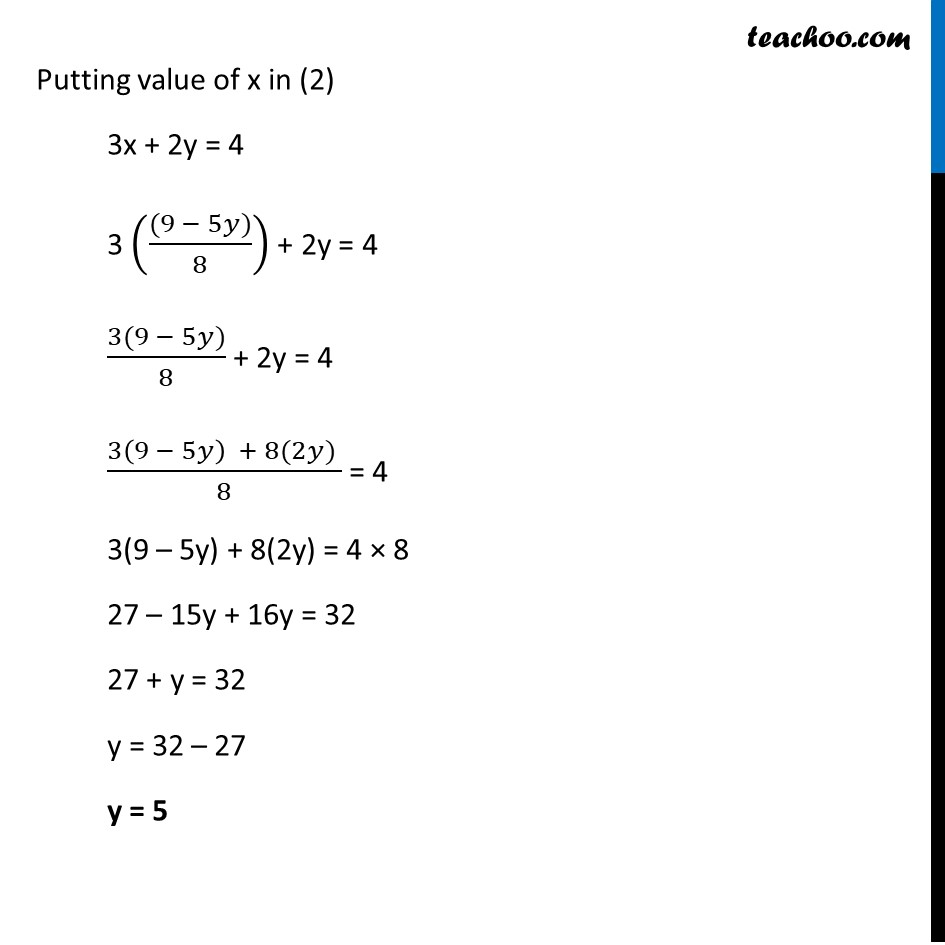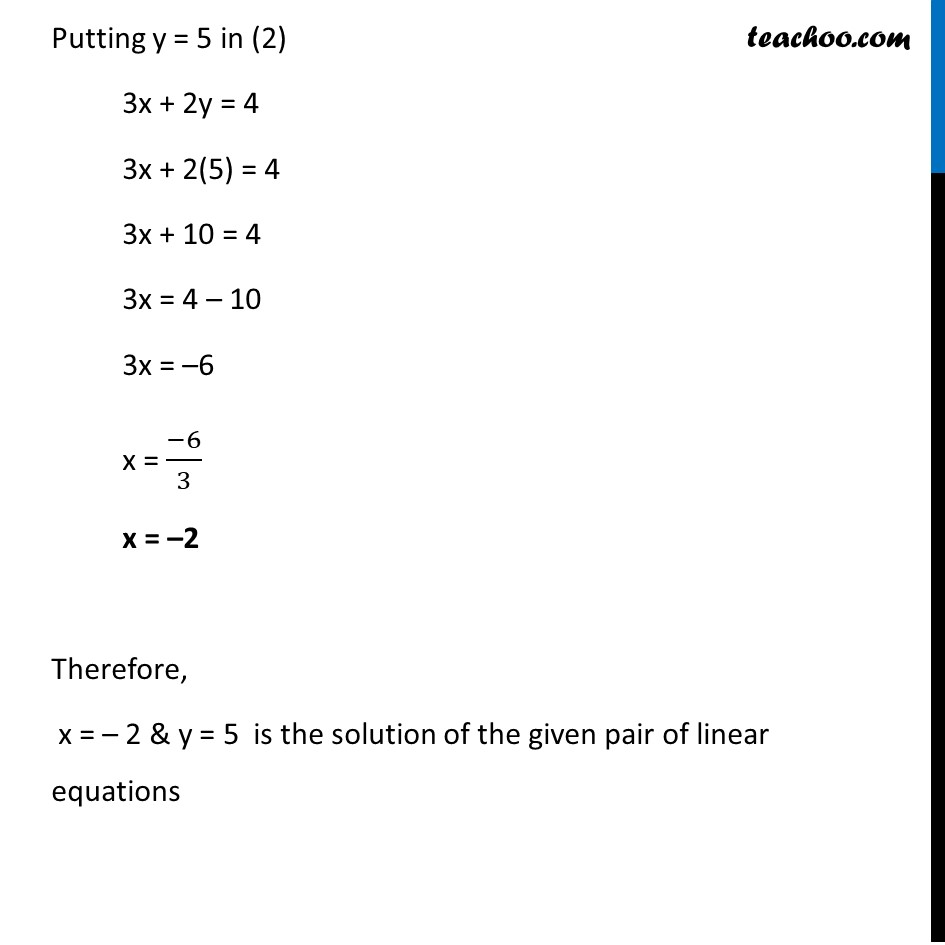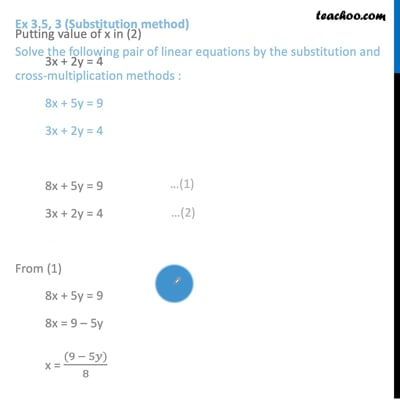This video is only available for Teachoo black users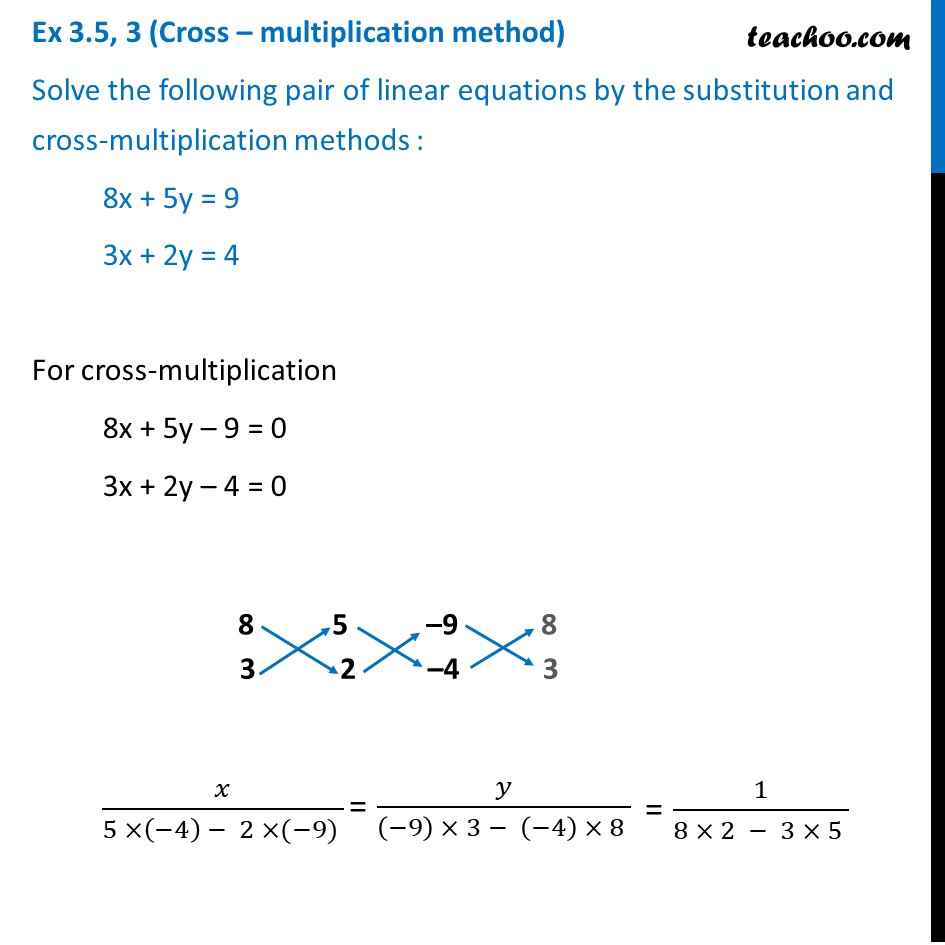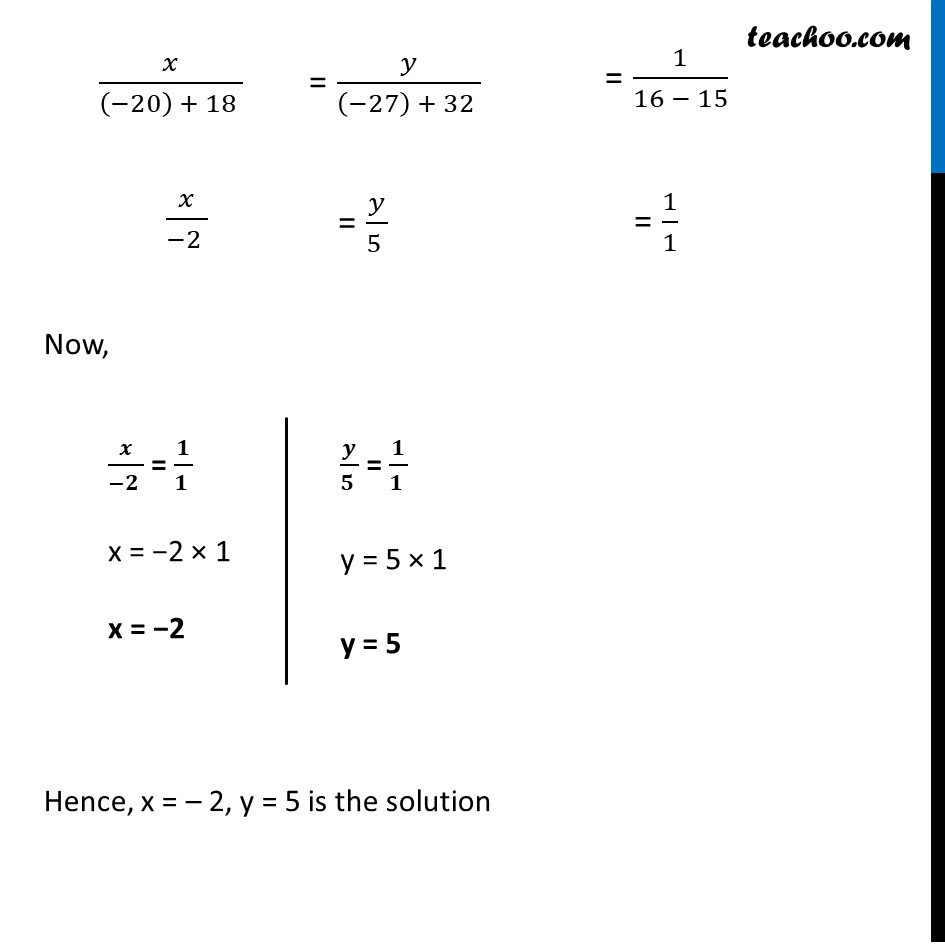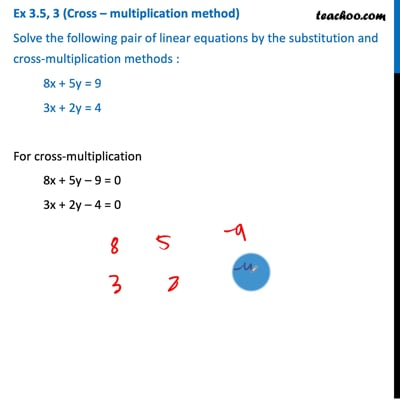This video is only available for Teachoo black users

Solve all your doubts with Teachoo Black (new monthly pack available now!)

### Transcript

Ex 3.5, 3 (Substitution method) Solve the following pair of linear equations by the substitution and cross-multiplication methods : 8x + 5y = 9 3x + 2y = 4 8x + 5y = 9 3x + 2y = 4 From (1) 8x + 5y = 9 8x = 9 – 5y x = ((9 − 5𝑦))/8 Putting value of x in (2) 3x + 2y = 4 3 (((9 − 5𝑦))/8) + 2y = 4 (3(9 − 5𝑦))/8 + 2y = 4 (3(9 − 5𝑦) + 8(2𝑦) )/8 = 4 3(9 – 5y) + 8(2y) = 4 × 8 27 – 15y + 16y = 32 27 + y = 32 y = 32 – 27 y = 5 Putting y = 5 in (2) 3x + 2y = 4 3x + 2(5) = 4 3x + 10 = 4 3x = 4 – 10 3x = –6 x = (−6)/3 x = –2 Therefore, x = – 2 & y = 5 is the solution of the given pair of linear equations Ex 3.5, 3 (Cross – multiplication method) Solve the following pair of linear equations by the substitution and cross-multiplication methods : 8x + 5y = 9 3x + 2y = 4 For cross-multiplication 8x + 5y – 9 = 0 3x + 2y – 4 = 0 𝑥/(5 ×(−4) − 2 ×(−9) ) = 𝑦/((−9) × 3 − (−4) × 8 ) = 1/(8 × 2 − 3 × 5 ) 𝑥/((−20) + 18 ) = 𝑦/((−27) + 32 ) = 1/(16 − 15) 𝑥/(−2 ) = 𝑦/(5 ) = 1/1 Now, Hence, x = – 2, y = 5 is the solution